Chemical Reactions Chemical reactions are described by chemical

• Slides: 64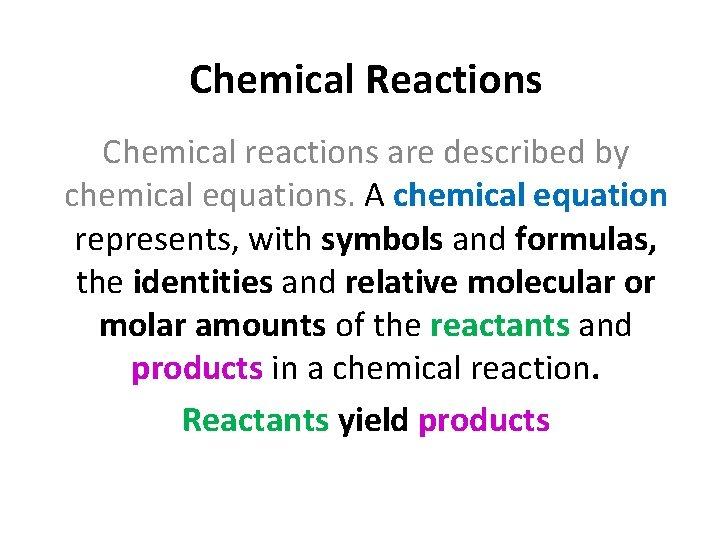Chemical Reactions Chemical reactions are described by chemical equations. A chemical equation represents, with symbols and formulas, the identities and relative molecular or molar amounts of the reactants and products in a chemical reaction. Reactants yield products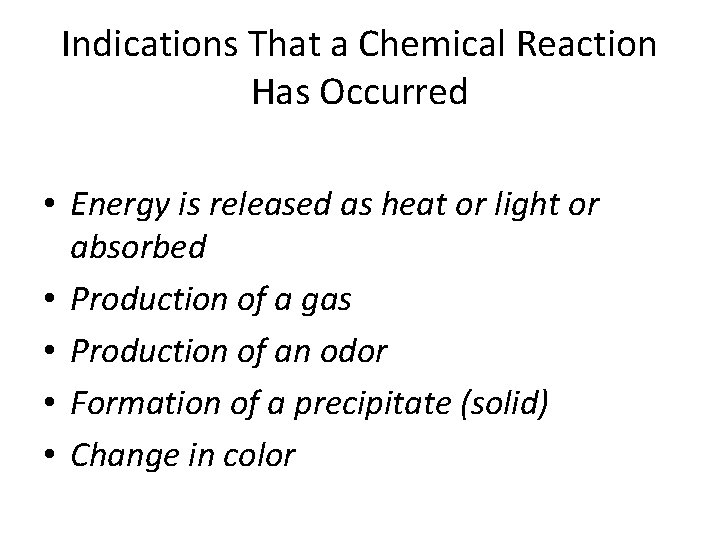Indications That a Chemical Reaction Has Occurred • Energy is released as heat or light or absorbed • Production of a gas • Production of an odor • Formation of a precipitate (solid) • Change in colorChemical Equations • We will start exploring chemical equations by expressing the reactants and products of chemical reactions in WORDS. • Next we will learn to express the reactants and products of chemical reactions with FORMULA (SKELETON) equations which use chemical formulas and symbols. • Lastly we will learn how to BALANCE chemical equations by placing COEFFICIENTS in front of chemical formulas so that the law of conservation of mass has been satisfied.Chemical Equations: Word Equations A word equation is an equation in which the reactants and products in a chemical reaction are represented by words. Such an equation has only qualitative meaning, because it does not give the quantities of the reactants used or the products formed. Methane + oxygen → carbon dioxide + water We read the ‘+’ as ‘and’, ‘reacts with’, ‘plus’, etc. We read the arrow ‘→’ as ‘react to yield’ or ‘produce’ or ‘form’. “Methane and oxygen react to yield carbon dioxide and water. ”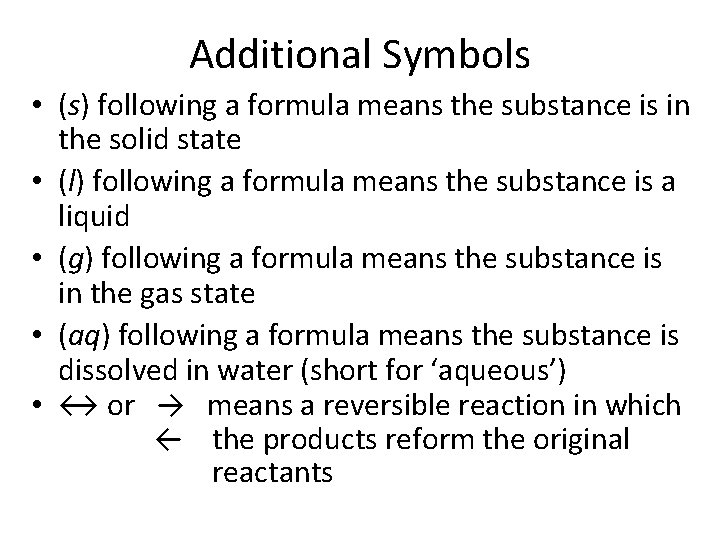Additional Symbols • (s) following a formula means the substance is in the solid state • (l) following a formula means the substance is a liquid • (g) following a formula means the substance is in the gas state • (aq) following a formula means the substance is dissolved in water (short for ‘aqueous’) • ↔ or → means a reversible reaction in which ← the products reform the original reactants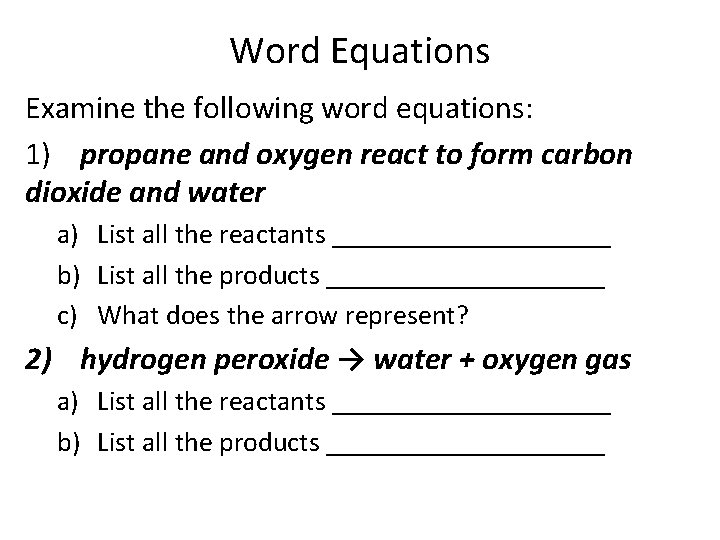Word Equations Examine the following word equations: 1) propane and oxygen react to form carbon dioxide and water a) List all the reactants __________ b) List all the products __________ c) What does the arrow represent? 2) hydrogen peroxide → water + oxygen gas a) List all the reactants __________ b) List all the products __________Practice translating word equations In the following word descriptions of chemical reactions, write the skeleton equation for each. Use your list of polyatomic ions and the notes on rules for naming compounds. Example: Calcium chloride reacts with sodium sulfate to produce calcium sulfate and sodium chloride. Ca. Cl 2 + Na 2 SO 4 → Ca. SO 4 + Na. Cl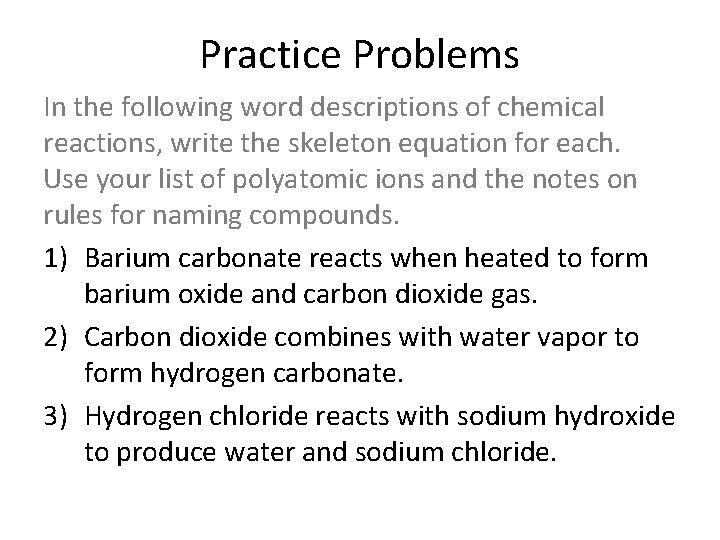Practice Problems In the following word descriptions of chemical reactions, write the skeleton equation for each. Use your list of polyatomic ions and the notes on rules for naming compounds. 1) Barium carbonate reacts when heated to form barium oxide and carbon dioxide gas. 2) Carbon dioxide combines with water vapor to form hydrogen carbonate. 3) Hydrogen chloride reacts with sodium hydroxide to produce water and sodium chloride.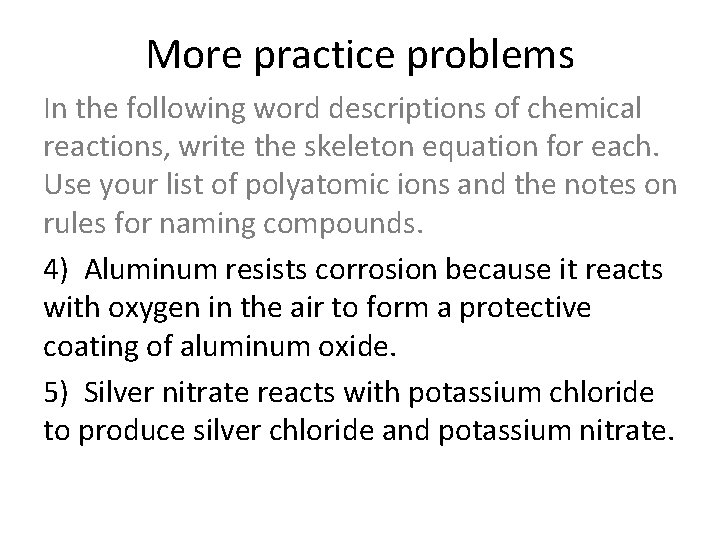More practice problems In the following word descriptions of chemical reactions, write the skeleton equation for each. Use your list of polyatomic ions and the notes on rules for naming compounds. 4) Aluminum resists corrosion because it reacts with oxygen in the air to form a protective coating of aluminum oxide. 5) Silver nitrate reacts with potassium chloride to produce silver chloride and potassium nitrate.More practice problems 6) Tin (IV) oxide reacts with sodium chloride to produce tin (IV) chloride plus sodium oxide 7) Iron (II) sulfate and aluminum chloride yield aluminum sulfate and iron (II) chloride 8) Rubidium carbonate and magnesium hydroxide produce rubidium hydroxide and magnesium carbonateCharacteristics of Chemical Equations 1. The equation must represent known facts. 2. The equation must contain the correct formulas for the reactants and the products. 3. The law of conservation of mass must be satisfied. A coefficient is a small whole number (integer) that appears in front of a formula in a chemical equation. The coefficient specifies the relative number of atoms or molecules or moles of that substance; if no number is written, the coefficient is assumed to be 1.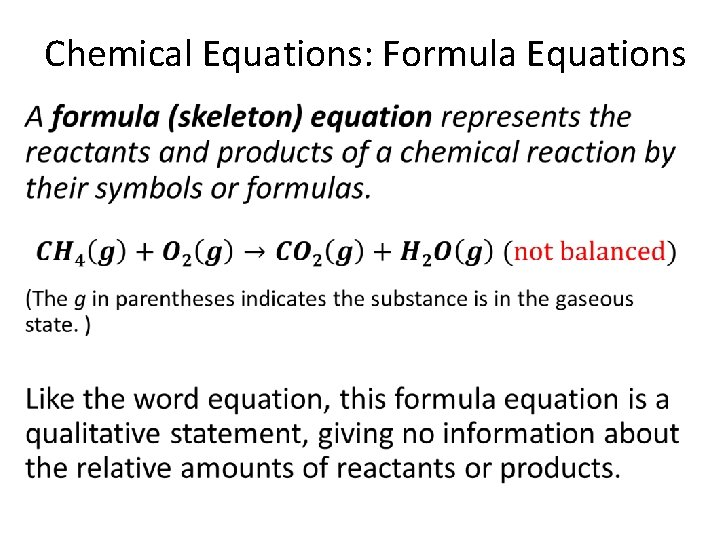Chemical Equations: Formula Equations •Balancing Chemical Equations • Reactants Elements # of atoms Products # of atoms 1 Carbon 1 4 Hydrogen 2 2 Oxygen 3Balancing Chemical Equations •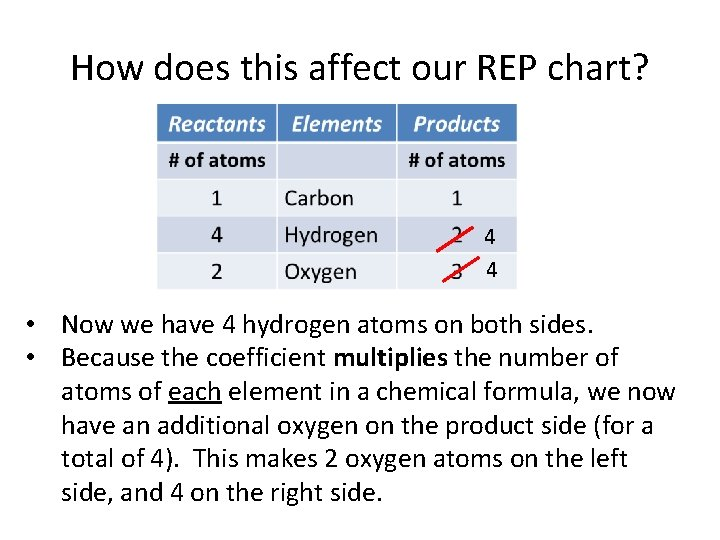How does this affect our REP chart? 4 4 • Now we have 4 hydrogen atoms on both sides. • Because the coefficient multiplies the number of atoms of each element in a chemical formula, we now have an additional oxygen on the product side (for a total of 4). This makes 2 oxygen atoms on the left side, and 4 on the right side.Balancing Chemical Equations • Hey! The number of atoms of each element are the same, so the equation is BALANCED!! 4 4 4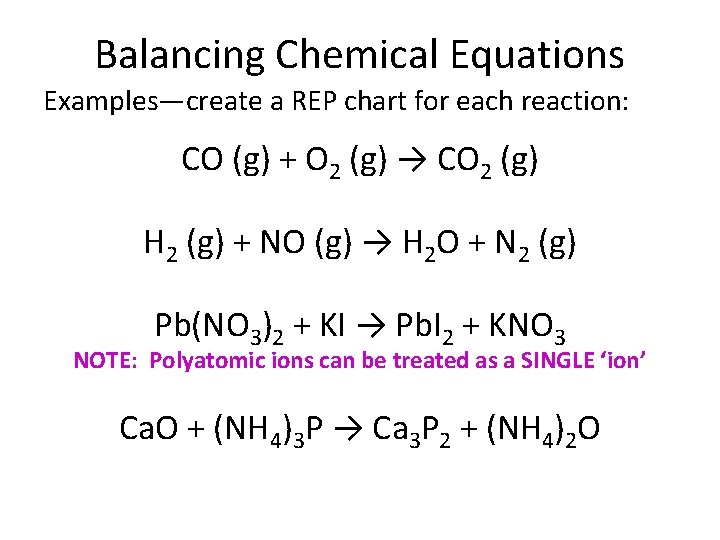Balancing Chemical Equations Examples—create a REP chart for each reaction: CO (g) + O 2 (g) → CO 2 (g) H 2 (g) + NO (g) → H 2 O + N 2 (g) Pb(NO 3)2 + KI → Pb. I 2 + KNO 3 NOTE: Polyatomic ions can be treated as a SINGLE ‘ion’ Ca. O + (NH 4)3 P → Ca 3 P 2 + (NH 4)2 O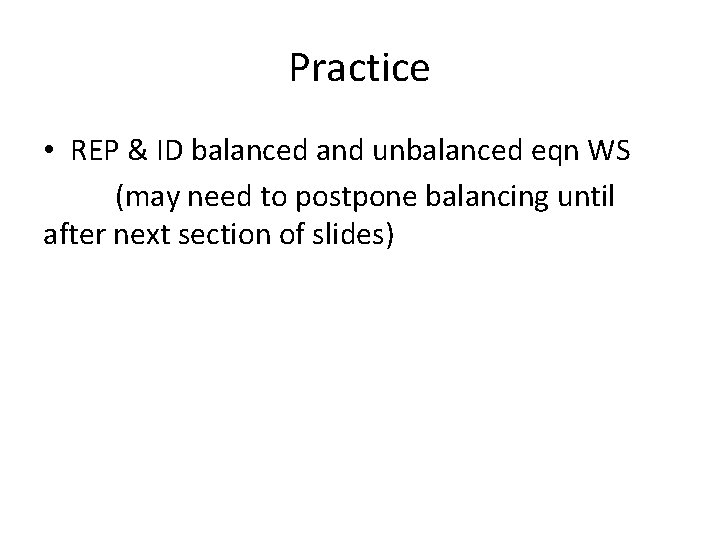Practice • REP & ID balanced and unbalanced eqn WS (may need to postpone balancing until after next section of slides)Rules for Balancing Chemical Reactions 1. Write a formula (skeleton) equation by substituting the correct formulas for the names of the reactants and the products. 2. Balance the formula equation according to the law of conservation of mass. (You can start with an atom inventory/REP table until you become more familiar with the process. ) Balancing is done by trial and error, but it helps to use these guidelines:Guidelines for Balancing Equations • Balance the different types of atoms one at a time • First balance the atoms of elements that are combined and that appear only once on each side of the equation • Balance polyatomic ions that appear on both sides of the equation as single units. • Balance H atoms and O atoms after atoms of all other elements have been balanced.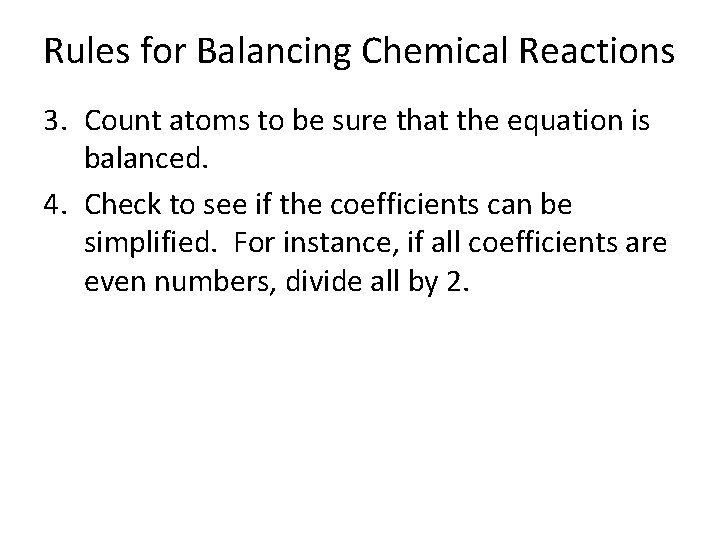Rules for Balancing Chemical Reactions 3. Count atoms to be sure that the equation is balanced. 4. Check to see if the coefficients can be simplified. For instance, if all coefficients are even numbers, divide all by 2.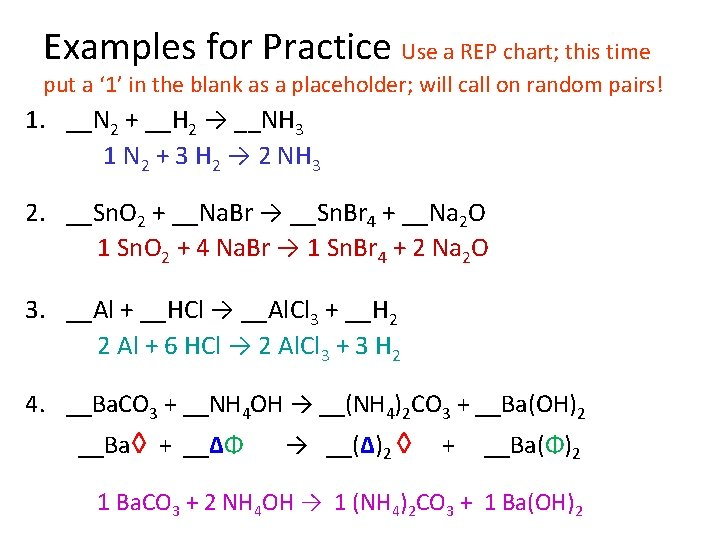Examples for Practice Use a REP chart; this time put a ‘ 1’ in the blank as a placeholder; will call on random pairs! 1. __N 2 + __H 2 → __NH 3 1 N 2 + 3 H 2 → 2 NH 3 2. __Sn. O 2 + __Na. Br → __Sn. Br 4 + __Na 2 O 1 Sn. O 2 + 4 Na. Br → 1 Sn. Br 4 + 2 Na 2 O 3. __Al + __HCl → __Al. Cl 3 + __H 2 2 Al + 6 HCl → 2 Al. Cl 3 + 3 H 2 4. __Ba. CO 3 + __NH 4 OH → __(NH 4)2 CO 3 + __Ba(OH)2 __Ba◊ + __∆Φ → __(∆)2 ◊ + __Ba(Φ)2 1 Ba. CO 3 + 2 NH 4 OH → 1 (NH 4)2 CO 3 + 1 Ba(OH)2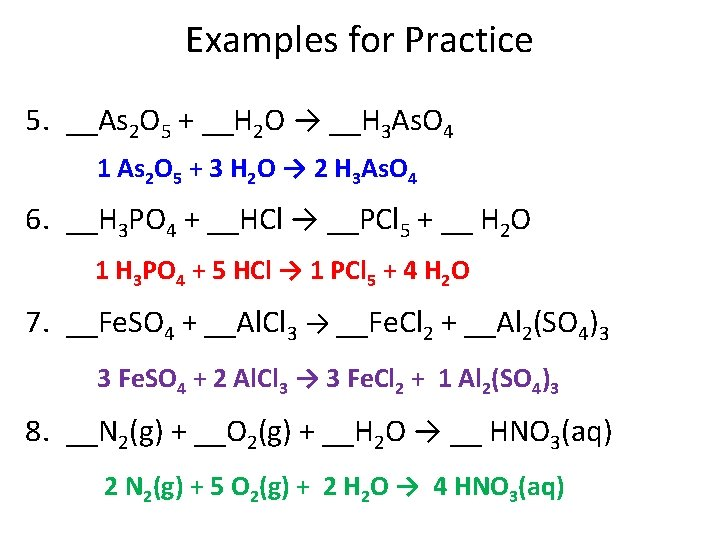Examples for Practice 5. __As 2 O 5 + __H 2 O → __H 3 As. O 4 1 As 2 O 5 + 3 H 2 O → 2 H 3 As. O 4 6. __H 3 PO 4 + __HCl → __PCl 5 + __ H 2 O 1 H 3 PO 4 + 5 HCl → 1 PCl 5 + 4 H 2 O 7. __Fe. SO 4 + __Al. Cl 3 → __Fe. Cl 2 + __Al 2(SO 4)3 3 Fe. SO 4 + 2 Al. Cl 3 → 3 Fe. Cl 2 + 1 Al 2(SO 4)3 8. __N 2(g) + __O 2(g) + __H 2 O → __ HNO 3(aq) 2 N 2(g) + 5 O 2(g) + 2 H 2 O → 4 HNO 3(aq)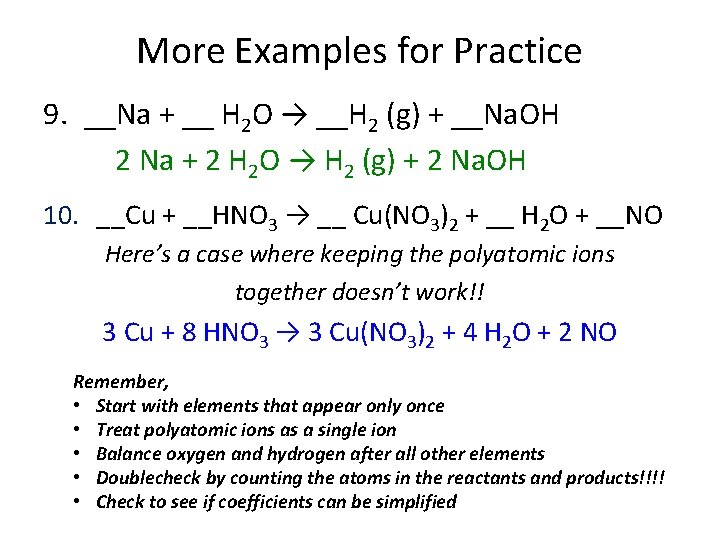More Examples for Practice 9. __Na + __ H 2 O → __H 2 (g) + __Na. OH 2 Na + 2 H 2 O → H 2 (g) + 2 Na. OH 10. __Cu + __HNO 3 → __ Cu(NO 3)2 + __ H 2 O + __NO Here’s a case where keeping the polyatomic ions together doesn’t work!! 3 Cu + 8 HNO 3 → 3 Cu(NO 3)2 + 4 H 2 O + 2 NO Remember, • Start with elements that appear only once • Treat polyatomic ions as a single ion • Balance oxygen and hydrogen after all other elements • Doublecheck by counting the atoms in the reactants and products!!!! • Check to see if coefficients can be simplifiedTypes of Chemical Reactions All chemical reactions can be placed into one of six categories. Here they are, in no particular order: 1) Combustion: A combustion reaction is when oxygen combines with another compound to form water and carbon dioxide. These reactions are exothermic, meaning they produce heat. An example of this kind of reaction is the burning of napthalene: C 10 H 8 + 12 O 2 ---> 10 CO 2 + 4 H 2 O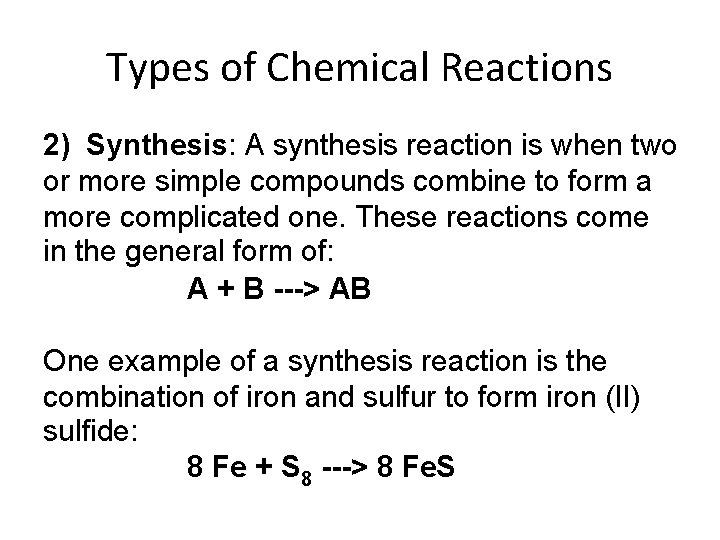Types of Chemical Reactions 2) Synthesis: A synthesis reaction is when two or more simple compounds combine to form a more complicated one. These reactions come in the general form of: A + B ---> AB One example of a synthesis reaction is the combination of iron and sulfur to form iron (II) sulfide: 8 Fe + S 8 ---> 8 Fe. SMore on Chemical Reactions • SPECIFIC TYPES OF SYNTHESIS REACTIONS ØElements with OXYGEN and SULFUR 2 Mg(s) + O 2(g) → 2 Mg. O(s) 8 Ba(s) + S 8(s) → 8 Ba. S(s) S 8(s) + 8 O 2(g) → 8 SO 2(g) ØMETALS with HALOGENS 2 Na(s) + Cl 2(g) → 2 Na. Cl(s) ØElements with OXIDES Ca. O(s) + H 2 O(l) → Ca(OH)2(s) SO 2(g) + H 2 O(l) → H 2 SO 3(aq)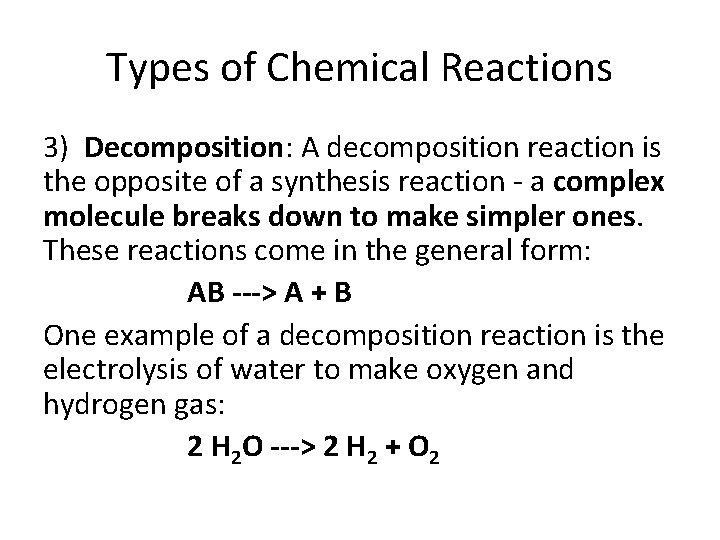Types of Chemical Reactions 3) Decomposition: A decomposition reaction is the opposite of a synthesis reaction - a complex molecule breaks down to make simpler ones. These reactions come in the general form: AB ---> A + B One example of a decomposition reaction is the electrolysis of water to make oxygen and hydrogen gas: 2 H 2 O ---> 2 H 2 + O 2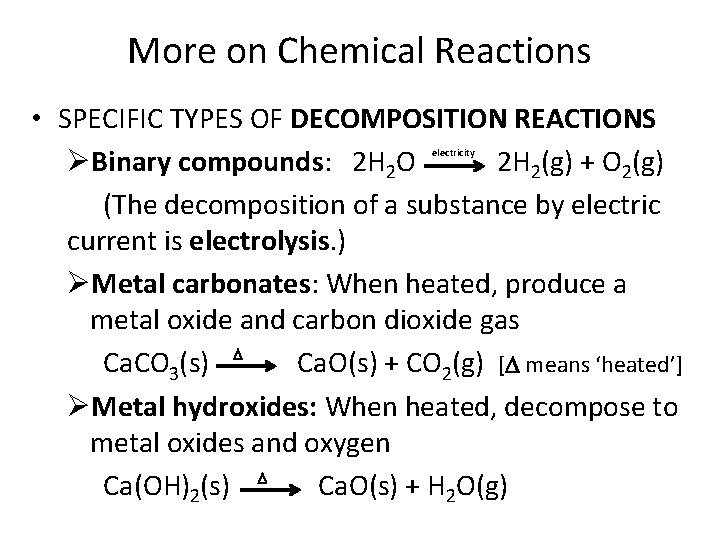More on Chemical Reactions • SPECIFIC TYPES OF DECOMPOSITION REACTIONS ØBinary compounds: 2 H 2 O 2 H 2(g) + O 2(g) (The decomposition of a substance by electric current is electrolysis. ) ØMetal carbonates: When heated, produce a metal oxide and carbon dioxide gas Ca. CO 3(s) Ca. O(s) + CO 2(g) [ means ‘heated’] ØMetal hydroxides: When heated, decompose to metal oxides and oxygen Ca(OH)2(s) Ca. O(s) + H 2 O(g) electricityMore decomposition reactions ØMetal chlorates: When heated, decompose to metal chlorides and oxygen (may need catalyst) 2 KCl. O 3(s) 2 KCl(s) + 3 O 2(g) ØAcids: Certain acids decompose into nonmetal oxides and water H 2 CO 3(aq) → CO 2(g) + H 2 O(l) Mn. O 2(s)Practice • Practice: Synthesis and decomp WS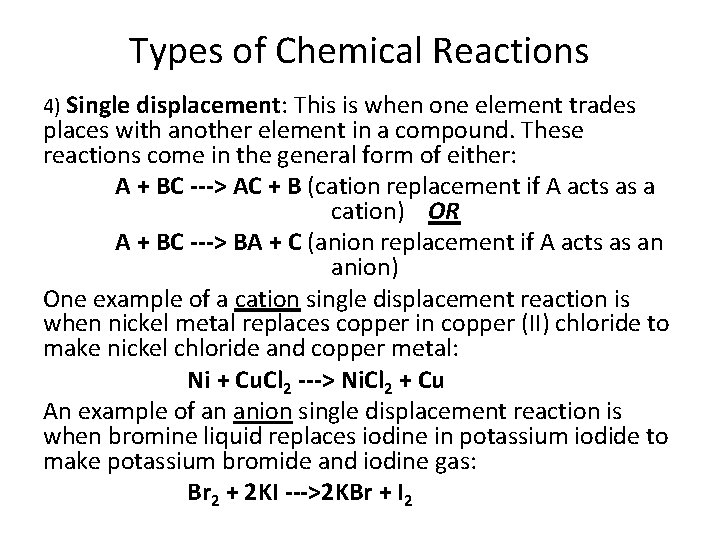Types of Chemical Reactions 4) Single displacement: This is when one element trades places with another element in a compound. These reactions come in the general form of either: A + BC ---> AC + B (cation replacement if A acts as a cation) OR A + BC ---> BA + C (anion replacement if A acts as an anion) One example of a cation single displacement reaction is when nickel metal replaces copper in copper (II) chloride to make nickel chloride and copper metal: Ni + Cu. Cl 2 ---> Ni. Cl 2 + Cu An example of an anion single displacement reaction is when bromine liquid replaces iodine in potassium iodide to make potassium bromide and iodine gas: Br 2 + 2 KI --->2 KBr + I 2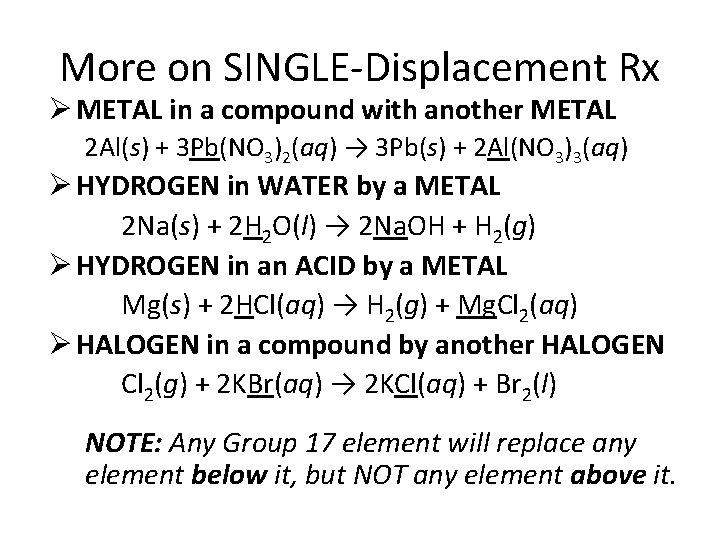More on SINGLE-Displacement Rx Ø METAL in a compound with another METAL 2 Al(s) + 3 Pb(NO 3)2(aq) → 3 Pb(s) + 2 Al(NO 3)3(aq) Ø HYDROGEN in WATER by a METAL 2 Na(s) + 2 H 2 O(l) → 2 Na. OH + H 2(g) Ø HYDROGEN in an ACID by a METAL Mg(s) + 2 HCl(aq) → H 2(g) + Mg. Cl 2(aq) Ø HALOGEN in a compound by another HALOGEN Cl 2(g) + 2 KBr(aq) → 2 KCl(aq) + Br 2(l) NOTE: Any Group 17 element will replace any element below it, but NOT any element above it.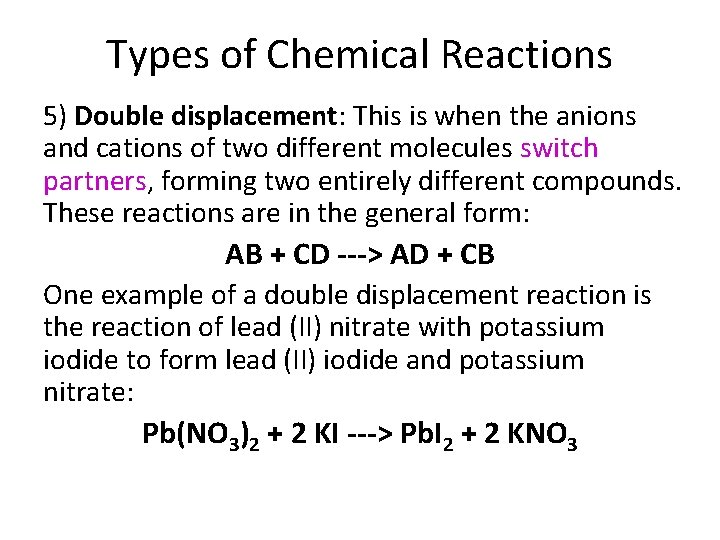Types of Chemical Reactions 5) Double displacement: This is when the anions and cations of two different molecules switch partners, forming two entirely different compounds. These reactions are in the general form: AB + CD ---> AD + CB One example of a double displacement reaction is the reaction of lead (II) nitrate with potassium iodide to form lead (II) iodide and potassium nitrate: Pb(NO 3)2 + 2 KI ---> Pb. I 2 + 2 KNO 3DOUBLE-Displacement Rx • The ions of 2 compounds exchange places in an aqueous solution to form 2 new compounds. One of the compounds is often soluble and remains dissolved; the other: Ø Forms a PRECIPITATE 2 KI(aq) + Pb(NO 3)2(aq) →Pb. I 2(s) + 2 KNO 3(aq) Ø Forms a GAS Fe. S(s) + 2 HCl(aq) → H 2 S(g) + Fe. Cl 2(aq) Ø Forms WATER HCl(aq) + Na. OH(aq) → Na. Cl(aq) + H 2 O(l)Types of Chemical Reactions 6) Acid-base: This is a special kind of double displacement reaction that takes place when an acid and base react with each other. The H+ ion in the acid reacts with the OH- ion in the base, causing the formation of water. Generally, the product of this reaction is some ionic salt and water: HA + BOH ---> H 2 O + BA One example of an acid-base reaction is the reaction of hydrobromic acid (HBr) with sodium hydroxide: HBr + Na. OH ---> Na. Br + H 2 O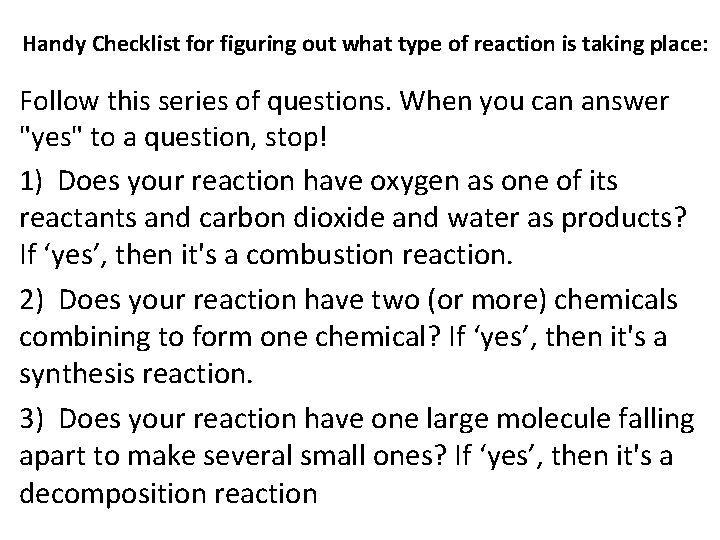Handy Checklist for figuring out what type of reaction is taking place: Follow this series of questions. When you can answer "yes" to a question, stop! 1) Does your reaction have oxygen as one of its reactants and carbon dioxide and water as products? If ‘yes’, then it's a combustion reaction. 2) Does your reaction have two (or more) chemicals combining to form one chemical? If ‘yes’, then it's a synthesis reaction. 3) Does your reaction have one large molecule falling apart to make several small ones? If ‘yes’, then it's a decomposition reaction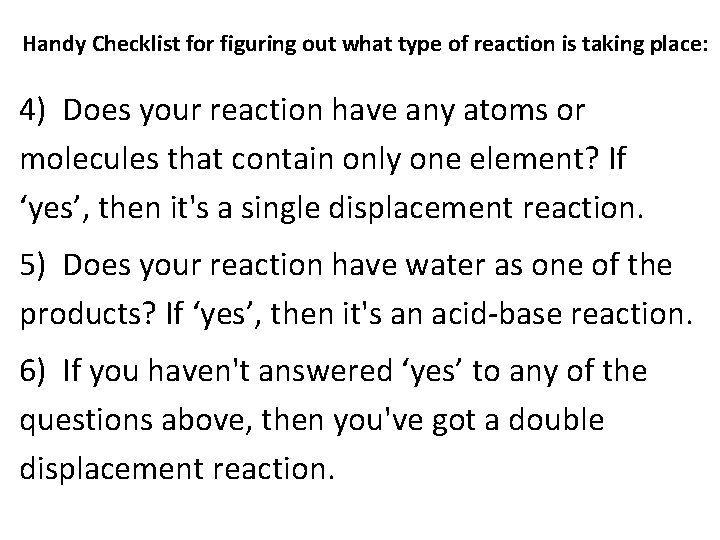Handy Checklist for figuring out what type of reaction is taking place: 4) Does your reaction have any atoms or molecules that contain only one element? If ‘yes’, then it's a single displacement reaction. 5) Does your reaction have water as one of the products? If ‘yes’, then it's an acid-base reaction. 6) If you haven't answered ‘yes’ to any of the questions above, then you've got a double displacement reaction.Types of Chemical Reactions • See handout and worksheets on 6 basic types of chemical reactions – Practice: S, D, SD, DD WS – Practice: A&B WS, C&D WSElements’ Reactivities Just because we can write a chemical equation does not necessarily mean that the reaction it shows will actually occur. Most metals do not occur in an uncombined, or “FREE” metallic state, because metals spontaneously react with oxygen in the atmosphere. Observing how readily a metal reacts with oxygen provides information about the metal’s chemical reactivity.What does ‘reactive’ mean? • Reactive means whether or not an element has a chemical reaction (precipitate, change in color, formation of a gas, or a change in temperature) • A more active metal will RELEASE a less active metal from its compounds. So if you put a more active metal like calcium together with a solution containing chromium ions, chromium metal will be formed and the calcium becomes ions in solutionCompare these 3 metals: • Copper metal at room temperature remains relatively unreactive with air but when heated combines with oxygen gas to make a black substance, copper oxide. • Magnesium metal when heated reacts with oxygen gas by igniting and producing a brief flash of light (magnesium oxide). • Gold does not react with oxygen gas (or any other components of air). RANK THEM FROM MOST REACTIVE TO LEAST REACTIVE Magnesium → Copper → Gold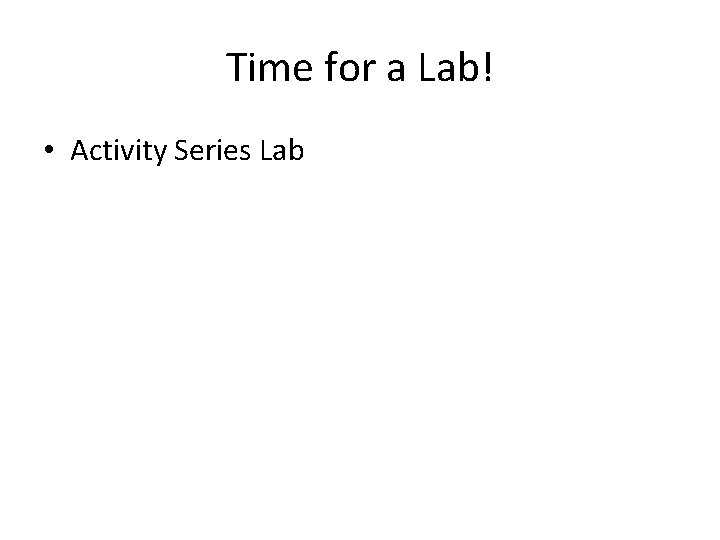Time for a Lab! • Activity Series LabPredicting Products of Chemical Rx An ACTIVITY SERIES is the ranking of elements in relative order of their chemical reactivities. • Activity series are used (1) to predict whether certain chemical reactions will take place and (2) what the products will be. • We have already mentioned the halogens Which is most reactive? Which is least reactive? • Metal activity is important in many reactions.Metal Activity Series Carbon and hydrogen are included for comparison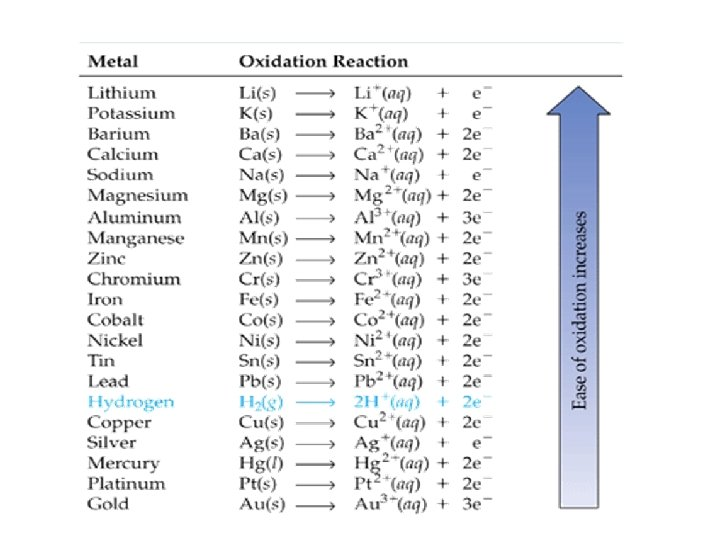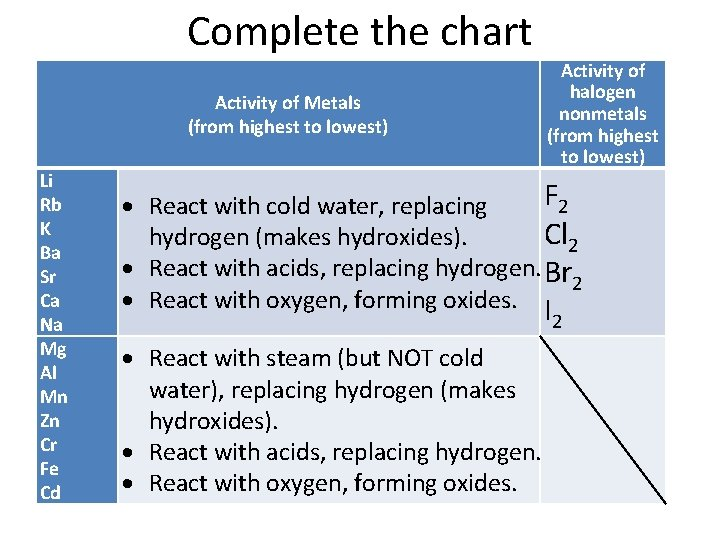Complete the chart Activity of Metals (from highest to lowest) Li Rb K Ba Sr Ca Na Mg Al Mn Zn Cr Fe Cd Activity of halogen nonmetals (from highest to lowest) F 2 React with cold water, replacing Cl 2 hydrogen (makes hydroxides). React with acids, replacing hydrogen. Br 2 React with oxygen, forming oxides. I 2 React with steam (but NOT cold water), replacing hydrogen (makes hydroxides). React with acids, replacing hydrogen. React with oxygen, forming oxides.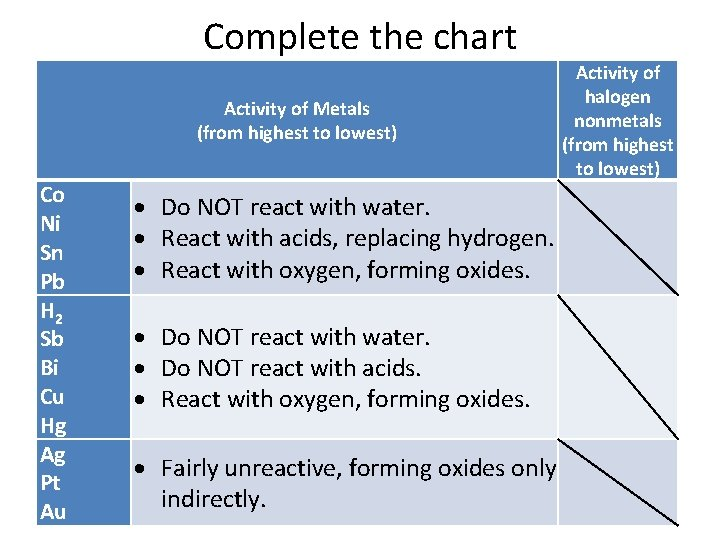Complete the chart Activity of Metals (from highest to lowest) Co Ni Sn Pb H 2 Sb Bi Cu Hg Ag Pt Au Do NOT react with water. React with acids, replacing hydrogen. React with oxygen, forming oxides. Do NOT react with water. Do NOT react with acids. React with oxygen, forming oxides. Activity of halogen nonmetals (from highest to lowest) Fairly unreactive, forming oxides only indirectly.Helpful hint Activity of halogen nonmetals (highest to lowest) Activity of Metals (highest to lowest) Replaces metal below it Li Rb K Ba Sr Ca Na Mg Al Mn Zn Cr Fe Cd Co Ni Sn Pb H 2 Sb Bi Cu Hg Ag Pt Au React with cold water, replacing hydrogen (makes hydroxides). React with acids, replacing hydrogen. React with oxygen, forming oxides. React with steam (but NOT cold water), replacing hydrogen (makes hydroxides). React with acids, replacing hydrogen. React with oxygen, forming oxides. Do NOT react with water. Do NOT react with acids. React with oxygen, forming oxides. Fairly unreactive, forming oxides only indirectly. F 2 Cl 2 Br 2 I 2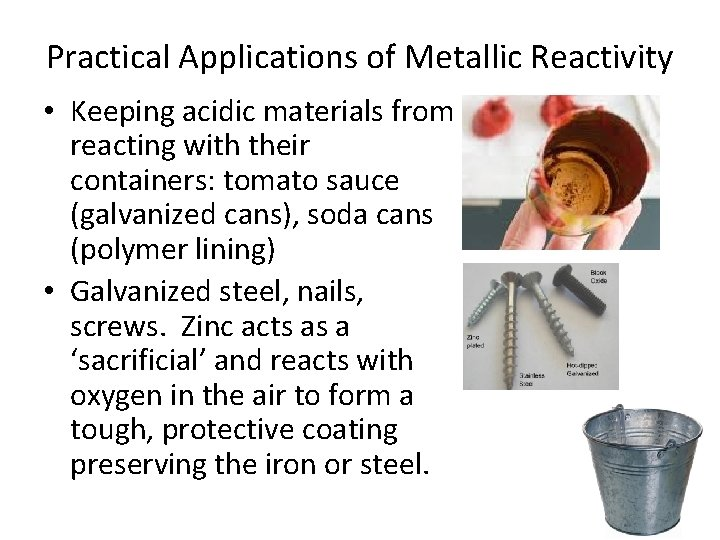Practical Applications of Metallic Reactivity • Keeping acidic materials from reacting with their containers: tomato sauce (galvanized cans), soda cans (polymer lining) • Galvanized steel, nails, screws. Zinc acts as a ‘sacrificial’ and reacts with oxygen in the air to form a tough, protective coating preserving the iron or steel.So how do we get ‘free’ metals? Most metals are not found free in nature; they are found combined with other elements in more stable compounds (ores). These compounds must be mined and refined to produce the free metal.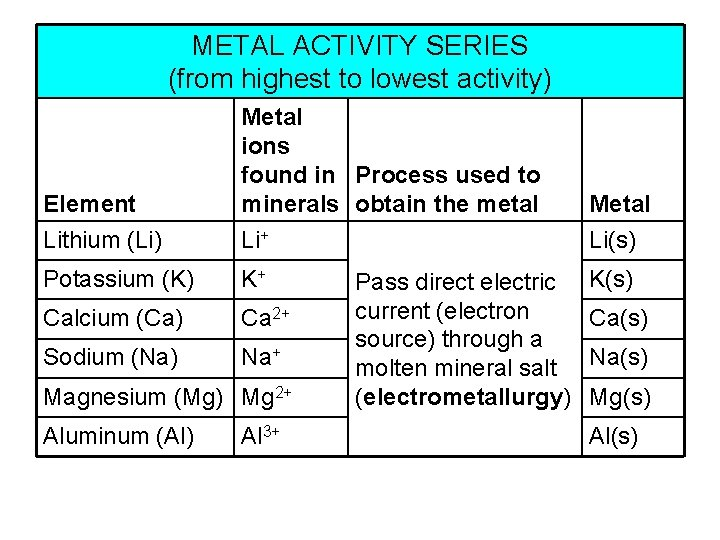METAL ACTIVITY SERIES (from highest to lowest activity) Element Metal ions found in Process used to minerals obtain the metal Metal Lithium (Li) Li+ Li(s) Potassium (K) K+ Calcium (Ca) Ca 2+ Sodium (Na) Na+ Magnesium (Mg) Mg 2+ Aluminum (Al) Al 3+ Pass direct electric current (electron source) through a molten mineral salt (electrometallurgy) K(s) Ca(s) Na(s) Mg(s) Al(s)METAL ACTIVITY SERIES (CONTINUED) (in order of decreasing activity) Element Metal ions found in Process used to minerals obtain the metal Manganese (Mn) Mn 3+ Mn 2+, Zinc (Zn) Zn 2+ Chromium (Cr) Cr 3+, Cr 2+ Iron (Fe) Fe 3+, Fe 2+ Roast or heat mineral in air with coke (C) or carbon monoxide (CO) (electron sources) Metal Mn(s) Zn(s) Cr(s) (pyrometallurgy) Fe(s)METAL ACTIVITY SERIES (CONTINUED) (in order of decreasing activity) Element Metal ions Process used to found in minerals obtain the metal Metal Lead (Pb) Pb Pb(s) Copper (Cu) Cu 2+, Mercury (Hg) Hg 2+ Silver (Ag) Ag+ Platinum (Pt) Pt 2+ Gold (Au) Au 3+, Au+ Cu+ Pyrometallurgy or treat ores and other metal-containing materials (tailings— mined rock leftover after leaching has removed most of the desired mineral) with reactants in a water solution (hydrometallurgy) Cu(s) Hg(s) Ag(s) Pt(s) Au(s)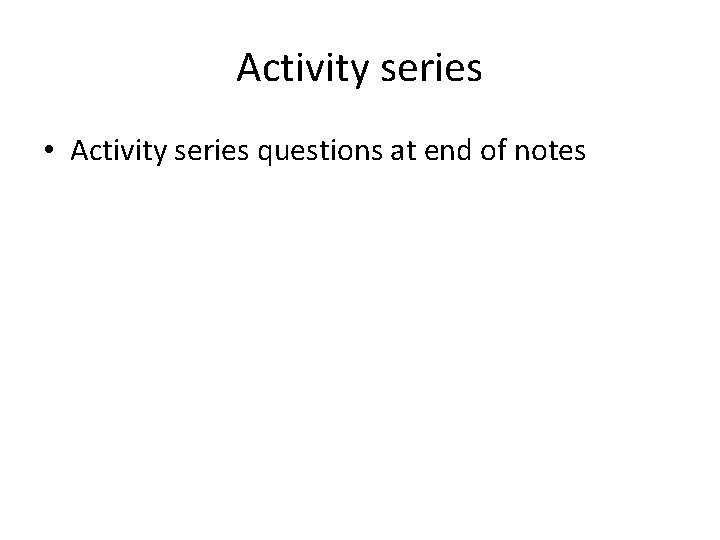Activity series • Activity series questions at end of notesSo how do we get ‘free’ metals? The process of converting a combined metal (usually a metal ion) in a mineral to a free metal involves a particular kind of chemical change, what we call a redox reaction.For instance, converting a copper (II) cation to an atom of copper metal requires adding two electrons. (The electrons appear on the reactant side of the reaction. ) Cu 2+ + 2 e– → Cu A chemical change in which a reactant GAINS one or more electrons is a REDUCTION reaction. The reverse reaction, in which an atom LOSES one or more electrons, is an OXIDATION reaction. (Notice that the electrons are on the product side of the reaction. ) Cu → Cu 2+ + 2 e–Confusing, huh? You need an mnemonic to help you remember! OILRIG Oxidation Is Loss [of electrons] Reduction Is Gain [of electrons]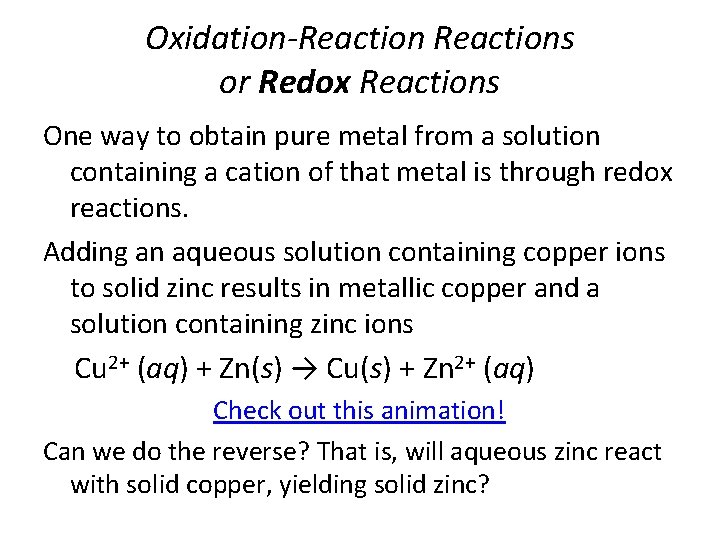Oxidation-Reactions or Redox Reactions One way to obtain pure metal from a solution containing a cation of that metal is through redox reactions. Adding an aqueous solution containing copper ions to solid zinc results in metallic copper and a solution containing zinc ions Cu 2+ (aq) + Zn(s) → Cu(s) + Zn 2+ (aq) Check out this animation! Can we do the reverse? That is, will aqueous zinc react with solid copper, yielding solid zinc?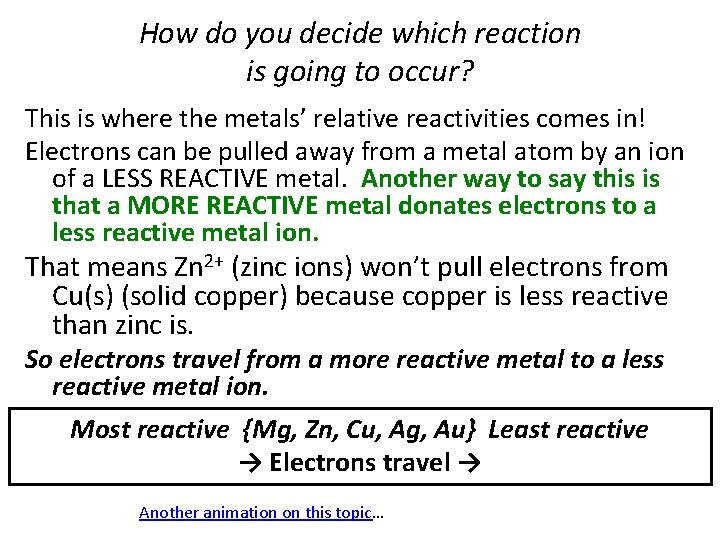How do you decide which reaction is going to occur? This is where the metals’ relative reactivities comes in! Electrons can be pulled away from a metal atom by an ion of a LESS REACTIVE metal. Another way to say this is that a MORE REACTIVE metal donates electrons to a less reactive metal ion. That means Zn 2+ (zinc ions) won’t pull electrons from Cu(s) (solid copper) because copper is less reactive than zinc is. So electrons travel from a more reactive metal to a less reactive metal ion. Most reactive {Mg, Zn, Cu, Ag, Au} Least reactive → Electrons travel → Another animation on this topic…Consider this metal activity series: Most reactive {Mg, Zn, Cu, Ag, Au} Least reactive → Electrons travel → Which one of these reactions will occur? 2 Ag+ (aq) + Mg(s) → 2 Ag(s) + Mg 2+ (aq) OR Mg 2+ (aq) + 2 Ag(s) → 2 Ag+ (aq) + Mg(s) Since electrons travel from the more reactive metal atom to the less reactive metal ion, the FIRST reaction will occur. (Magnesium atom donates electrons to the silver ion because magnesium is more reactive than silver. )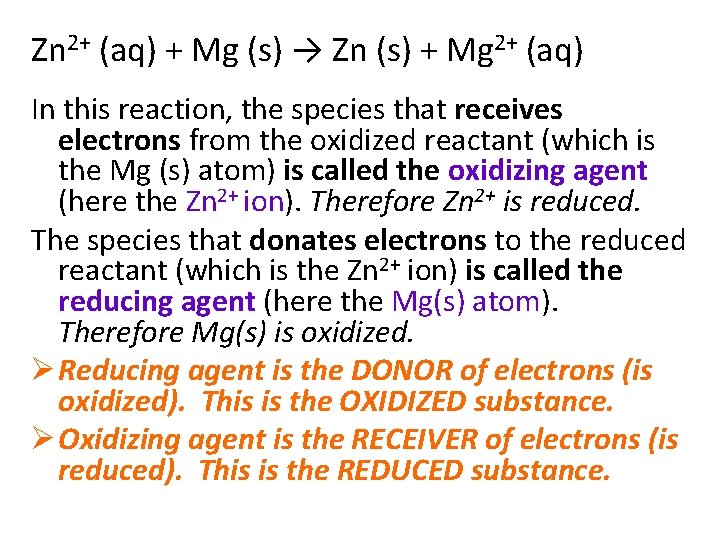Zn 2+ (aq) + Mg (s) → Zn (s) + Mg 2+ (aq) In this reaction, the species that receives electrons from the oxidized reactant (which is the Mg (s) atom) is called the oxidizing agent (here the Zn 2+ ion). Therefore Zn 2+ is reduced. The species that donates electrons to the reduced reactant (which is the Zn 2+ ion) is called the reducing agent (here the Mg(s) atom). Therefore Mg(s) is oxidized. Ø Reducing agent is the DONOR of electrons (is oxidized). This is the OXIDIZED substance. Ø Oxidizing agent is the RECEIVER of electrons (is reduced). This is the REDUCED substance.Half-Reactions Zn 2+ + 2 e- → Zn(s) Electrons GAINED Reduction Mg(s) → Mg 2+ + 2 e- Mg(s) − 2 e- → Mg 2+ The convention in chemistry is to show oxidation with the electrons lost on the PRODUCT side of the reaction (black font above). Electrons LOST Oxidation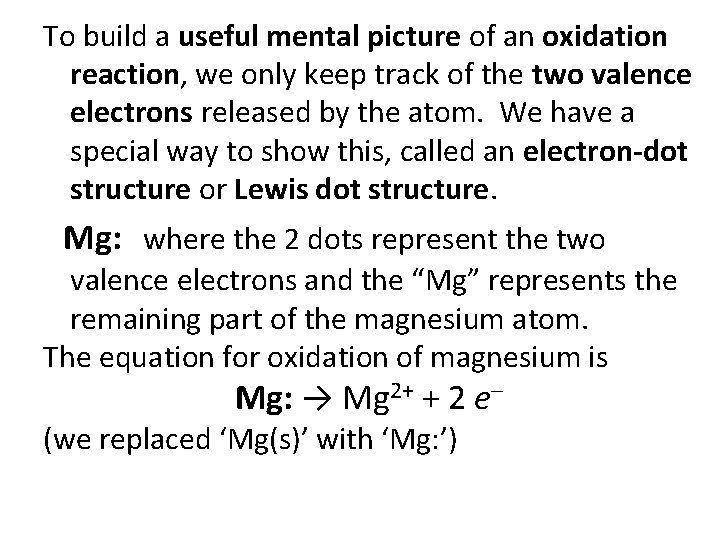To build a useful mental picture of an oxidation reaction, we only keep track of the two valence electrons released by the atom. We have a special way to show this, called an electron-dot structure or Lewis dot structure. Mg: where the 2 dots represent the two valence electrons and the “Mg” represents the remaining part of the magnesium atom. The equation for oxidation of magnesium is Mg: → Mg 2+ + 2 e– (we replaced ‘Mg(s)’ with ‘Mg: ’)